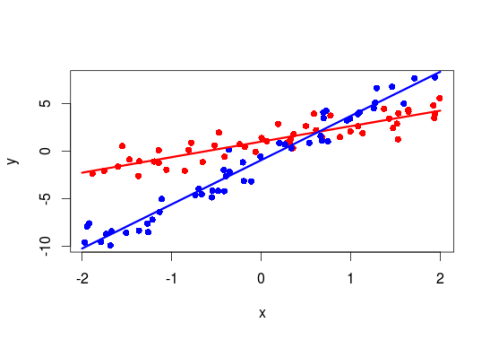Want to share your content on R-bloggers? click here if you have a blog, or here if you don't.

This is a pretty short post on an issue that popped at some point in the past, at that time I found a way around it but as it arose again recently I decided to go through it.

The issue I had was that when modeling an interaction between a continuous (say temperature) and a categorical variables (say site ID), we get the slope for the first level (the baseline) of the categorical variable and the difference between the remaining levels and this baseline slope. Sometime it is not only interesting to get if we have differences between the levels but also to know if the slopes at the different levels are different from 0.

Let’s see how this works:

```set.seed(20160315)
#simulate some data
X<-data.frame(Temp=runif(100,-2,2),Site=gl(n=2,k=50))
#the model matrix
mm<-model.matrix(~Temp*Site,X)
#the coefficients for the models
bs<-c(1,1.5,-2,3)
#simulate the response
X\$y<-rnorm(100,mean=mm%*%bs,sd=1)
#fit the model
m<-lm(y~Temp*Site,X)
#summary table
summary(m)

Call:
lm(formula = y ~ Temp * Site, data = X)

Residuals:
Min      1Q  Median      3Q     Max
-2.2194 -0.6776 -0.1545  0.5517  2.7618

Coefficients:
Estimate Std. Error t value Pr(>|t|)
(Intercept)   0.9904     0.1474   6.717 1.31e-09 ***
Temp          1.6274     0.1280  12.715  < 2e-16 ***
Site2        -1.9410     0.2083  -9.319 4.33e-15 ***
Temp:Site2    3.0109     0.1842  16.343  < 2e-16 ***
---
Signif. codes:  0 ‘***’ 0.001 ‘**’ 0.01 ‘*’ 0.05 ‘.’ 0.1 ‘ ’ 1

Residual standard error: 1.031 on 96 degrees of freedom
Multiple R-squared:  0.943,	Adjusted R-squared:  0.9412
F-statistic: 528.9 on 3 and 96 DF,  p-value: < 2.2e-16

#a nice plot
new_x<-seq(-2,2,length=10)
plot(y~x,X,col=c("red","blue")[X\$Fac],pch=16)
lines(new_x,coef(m)["(Intercept)"]+coef(m)["x"]*new_x,col="red",lwd=3)
lines(new_x,(coef(m)["(Intercept)"]+coef(m)["Fac2"])+(coef(m)["x"]+coef(m)["x:Fac2"])*new_x,col="blue",lwd=3)
```From the summary table of this model we see that the slopes between y and Temp is significantly bigger in Site 2 compared to Site 1. But this does not tell us if the slope y~Temp is different from 0 in Site 2.

Getting the slope y~Temp in Site 2 is easy:

```#get the estimated slope for Fac2
b<-coef(m)+coef(m)
```

But we need some estimation of uncertainty (ie standard error) around this slope to get to a p-value. We can achieve this by knowing that:

Var(a+b) = Var(a) + Var(b) + 2*cov(a,b)

It is rather easy to get this information from a lm object in R, the `vcov` function return the variance-covariance matrix of the model coefficient. The diagonal values are the coefficient variances and the off-diagonal values are the covariances. The standard error is then the square root of the variance.

```#get the standard error for the slope of Fac2
se<-sqrt(vcov(m)["x","x"]+vcov(m)["x:Fac2","x:Fac2"]+2*vcov(m)["x","x:Fac2"])
#compute the two-sided p-values that the slope for Fac2 is different from 0
pt(b/se, df = nrow(X)-length(coef(m)),lower.tail=FALSE)*2
```

You can of course extend this to more than two-way interactions and for categorical variables with more than two levels. You just need to be careful when computing the different slopes and standard errors that you took all the appropriate coefficients.

Of course you may also fit separate models for each levels of your categorical variables, you should find the same results. The nice thing about fitting one model is that you get some indication about differences between the different levels which you would not get so easily from separate models …

Related Post